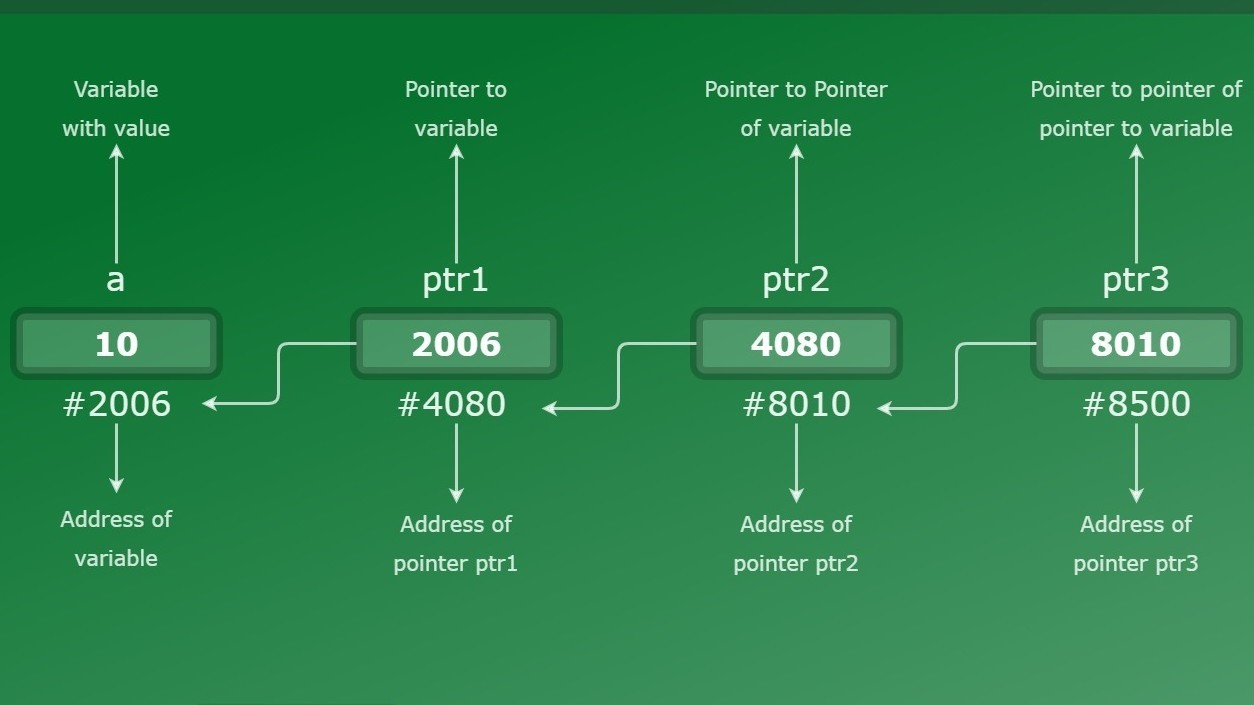Open In App

# Chain of Pointers in C with Examples

Prerequisite: Pointers in C, Double Pointer (Pointer to Pointer) in C
A pointer is used to point to a memory location of a variable. A pointer stores the address of a variable.
Similarly, a chain of pointers is when there are multiple levels of pointers. Simplifying, a pointer points to address of a variable, double-pointer points to a variable and so on. This is called multiple indirections.
Syntax:

```// level-1 pointer declaration
datatype *pointer;

// level-2 pointer declaration
datatype **pointer;

// level-3 pointer declaration
datatype ***pointer;
.
.
and so on```

The level of the pointer depends on how many asterisks the pointer variable is preceded with at the time of declaration.
Declaration:

```int *pointer_1;
int **pointer_2;
int ***pointer_3;
.
.
and so on```

Level of pointers or say chain can go up to N level depending upon the memory size. If you want to create a pointer of level-5, you need to precede the pointer variable name by 5 asterisks(*) at the time of declaration.
Initialization:

```// initializing level-1 pointer
// with address of variable 'var'
pointer_1 = &var;

// initializing level-2 pointer
// with address of level-1 pointer
pointer_2 = &pointer_1;

// initializing level-3 pointer
// with address of level-2 pointer
pointer_3 = &pointer_2;
.
.
and so on```

Example 1:As shown in the diagram, variable ‘a’ is a normal integer variable which stores integer value 10 and is at location 2006. ‘ptr1’ is a pointer variable which points to integer variable ‘a’ and stores its location i.e. 2006, as its value. Similarly ptr2 points to pointer variable ptr1 and ptr3 points at pointer variable ptr2. As every pointer is directly or indirectly pointing to the variable ‘a’, they all have the same integer value as variable ‘a’, i.e. 10
Let’s understand better with below given code:

## C

 `#include ``// C program for chain of pointer` `int` `main()``{``    ``int` `var = 10;` `    ``// Pointer level-1``    ``// Declaring pointer to variable var``    ``int``* ptr1;` `    ``// Pointer level-2``    ``// Declaring pointer to pointer variable *ptr1``    ``int``** ptr2;` `    ``// Pointer level-3``    ``// Declaring pointer to double pointer **ptr2``    ``int``*** ptr3;` `    ``// Storing address of variable var``    ``// to pointer variable ptr1``    ``ptr1 = &var;` `    ``// Storing address of pointer variable``    ``// ptr1 to level -2 pointer ptr2``    ``ptr2 = &ptr1;` `    ``// Storing address of level-2 pointer``    ``// ptr2 to level-3 pointer ptr3``    ``ptr3 = &ptr2;` `    ``// Displaying values``    ``printf``("Value of variable "``           ``"var = %d\n",``           ``var);``    ``printf``("Value of variable var ``using``"``           ``" pointer ptr1 = %d\n",``           ``*ptr1);``    ``printf``("Value of variable var ``using``"``           ``" pointer ptr2 = %d\n",``           ``**ptr2);``    ``printf``("Value of variable var ``using``"``           ``" pointer ptr3 = %d\n",``           ``***ptr3);` `    ``return` `0;``}`

Output:

```Value of variable var = 10
Value of variable var using pointer ptr1 = 10
Value of variable var using pointer ptr2 = 10
Value of variable var using pointer ptr3 = 10```

Example 2: Consider below-given code where we have taken float data type of the variable, so now we have to take same data type for the chain of pointers too. As the pointer and the variable, it is pointing to should have the same data type.

## C

 `#include ``int` `main()``{``    ``float` `var = 23.564327;` `    ``// Declaring pointer variables upto level_4``    ``float` `*ptr1, **ptr2, ***ptr3, ****ptr4;` `    ``// Initializing pointer variables``    ``ptr1 = &var;``    ``ptr2 = &ptr1;``    ``ptr3 = &ptr2;``    ``ptr4 = &ptr3;` `    ``// Printing values``    ``printf``("Value of var = %f\n", var);``    ``printf``("Value of var ``using` `level-1"``           ``" pointer = %f\n",``           ``*ptr1);``    ``printf``("Value of var ``using` `level-2"``           ``" pointer = %f\n",``           ``**ptr2);``    ``printf``("Value of var ``using` `level-3"``           ``" pointer = %f\n",``           ``***ptr3);``    ``printf``("Value of var ``using` `level-4"``           ``" pointer = %f\n",``           ``****ptr4);` `    ``return` `0;``}`

Output:

```Value of var = 23.564327
Value of var using level-1 pointer = 23.564327
Value of var using level-2 pointer = 23.564327
Value of var using level-3 pointer = 23.564327
Value of var using level-4 pointer = 23.564327```

Example 3: Updating variable using chained pointer
As we already know that a pointer points to address location of a variable so when we access the value of pointer it’ll point to the variable’s value. Now to update the value of variable, we can use any level of pointer as ultimately every pointer is directly or indirectly pointing to that variable only. It’ll directly change the value present at the address location of variable.

## C

 `#include ` `int` `main()``{``    ``// Initializing integer variable``    ``int` `var = 10;` `    ``// Declaring pointer variables upto level-3``    ``int` `*ptr1, **ptr2, ***ptr3;` `    ``// Initializing pointer variables``    ``ptr1 = &var;``    ``ptr2 = &ptr1;``    ``ptr3 = &ptr2;` `    ``// Printing values BEFORE updation``    ``printf``("Before:\n");``    ``printf``("Value of var = %d\n", var);``    ``printf``("Value of var ``using` `level-1"``           ``" pointer = %d\n",``           ``*ptr1);``    ``printf``("Value of var ``using` `level-2"``           ``" pointer = %d\n",``           ``**ptr2);``    ``printf``("Value of var ``using` `level-3"``           ``" pointer = %d\n",``           ``***ptr3);` `    ``// Updating var's value using level-3 pointer``    ``***ptr3 = 35;` `    ``// Printing values AFTER updation``    ``printf``("After:\n");``    ``printf``("Value of var = %d\n", var);``    ``printf``("Value of var ``using` `level-1"``           ``" pointer = %d\n",``           ``*ptr1);``    ``printf``("Value of var ``using` `level-2"``           ``" pointer = %d\n",``           ``**ptr2);``    ``printf``("Value of var ``using` `level-3"``           ``" pointer = %d\n",``           ``***ptr3);` `    ``return` `0;``}`

Output:

```Before:
Value of var = 10
Value of var using level-1 pointer = 10
Value of var using level-2 pointer = 10
Value of var using level-3 pointer = 10
After:
Value of var = 35
Value of var using level-1 pointer = 35
Value of var using level-2 pointer = 35
Value of var using level-3 pointer = 35```

Note: Level-N pointer can only be used to point level-(N-1) pointer. Except for Level-1 pointer. The level-1 pointer will always point to the variable.